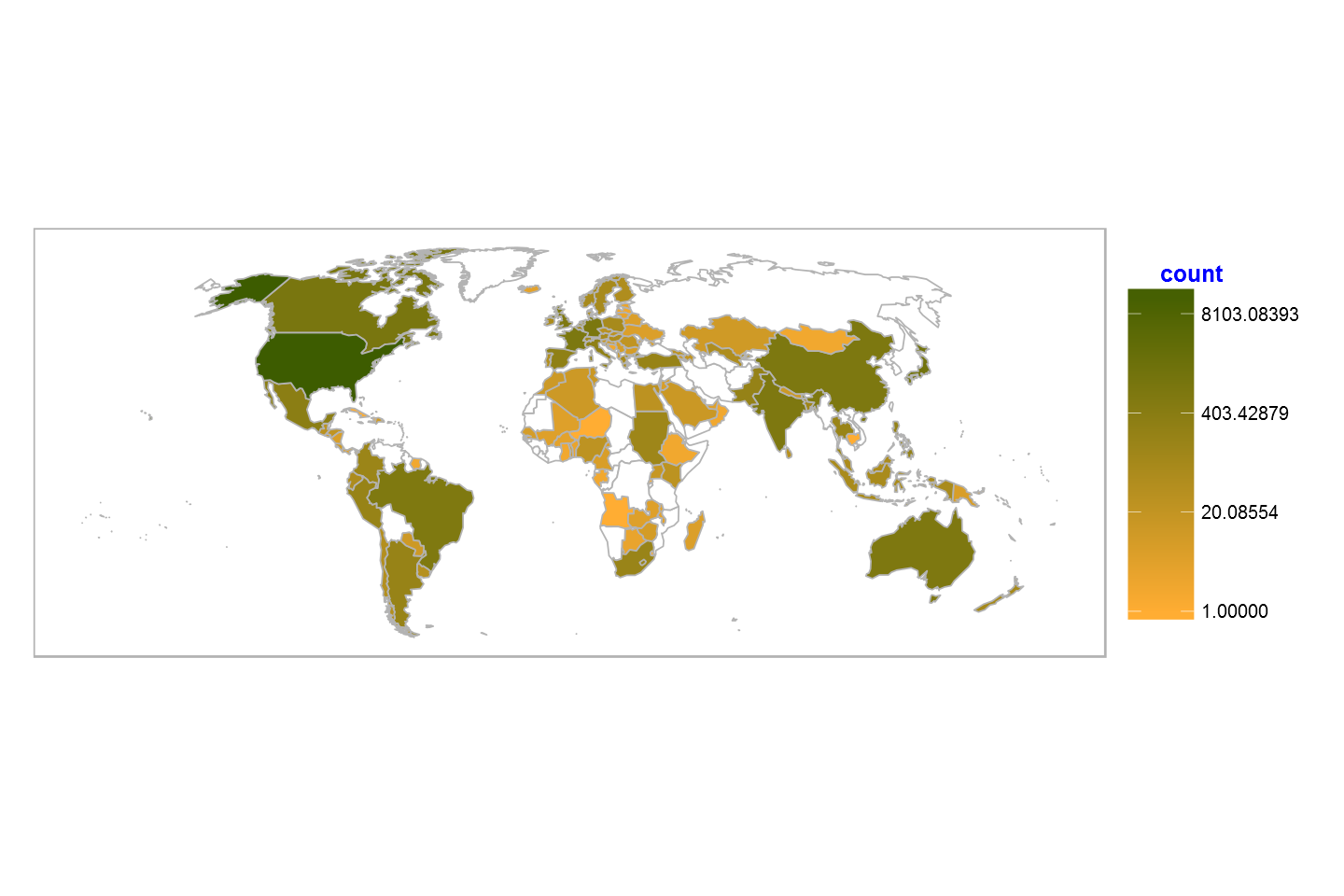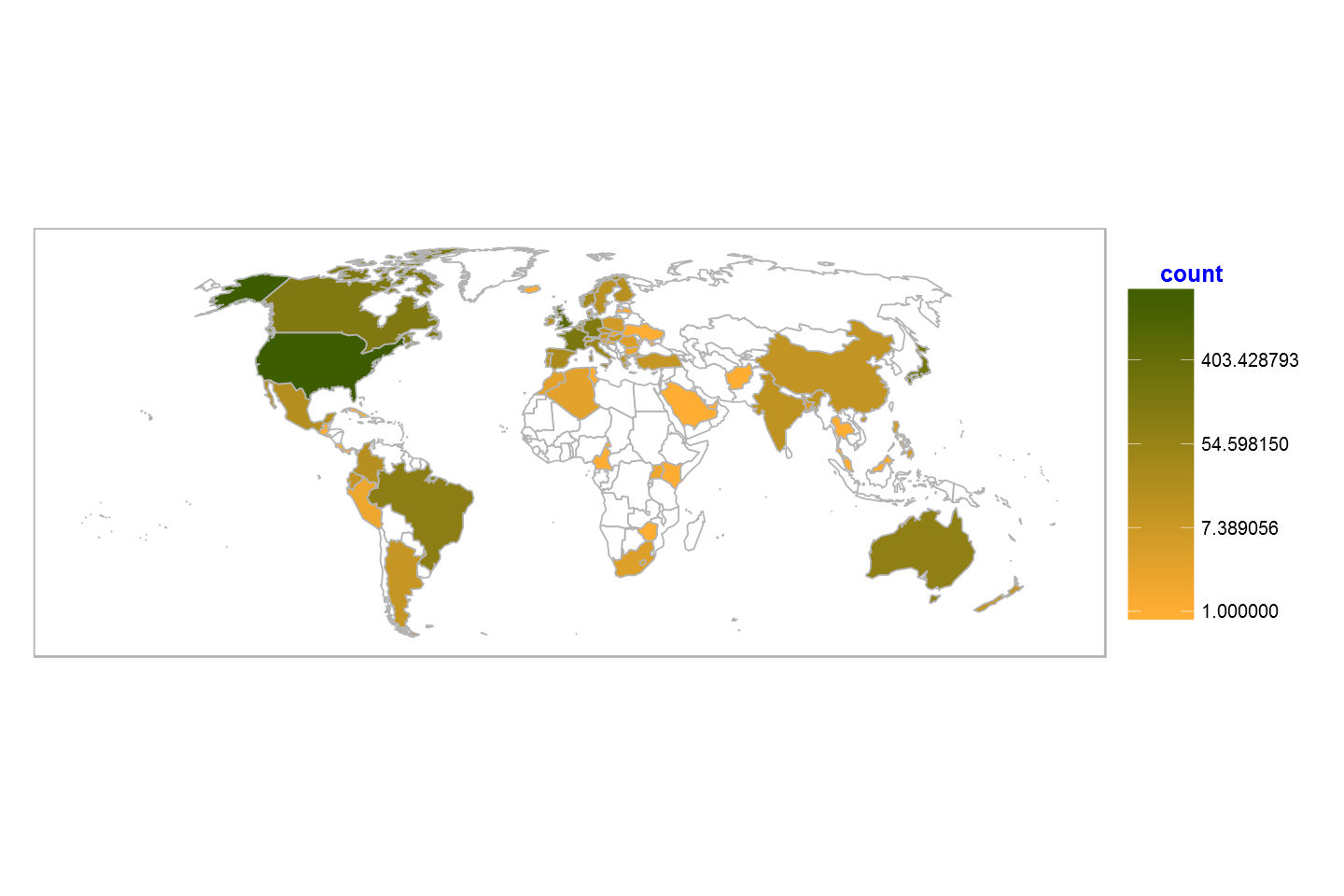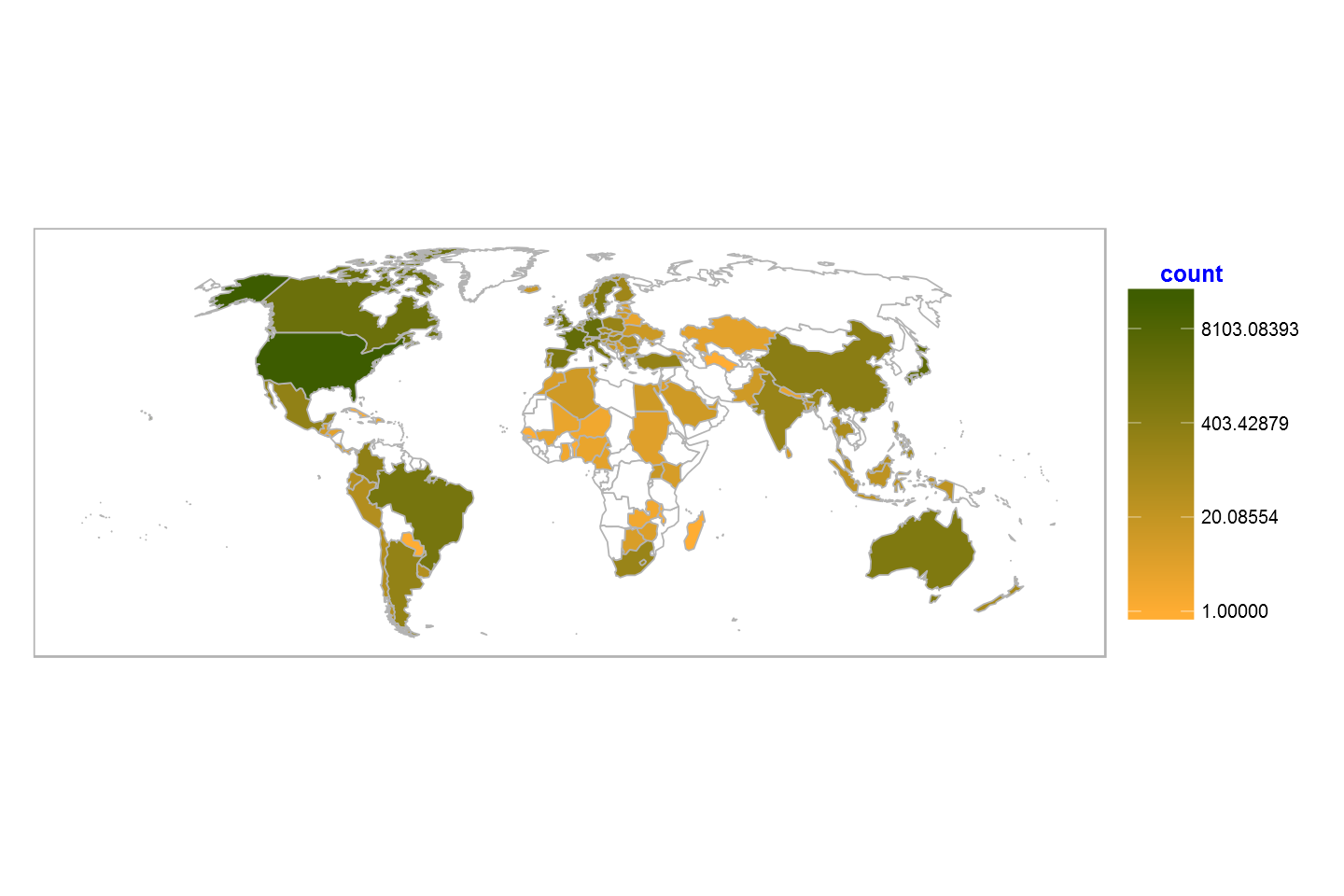# Fisseha Berhane, PhD

#### Data Scientist443-970-2353[email protected] CV Resume### Visualizing outcomes of drug related adverse events¶

How many of the drug related adverse events in the FDA database resulted in deaths, disabilities, hopspitalization, etc? To answer this question, we will download various datasets, join them, do some analysis and produce maps.

In :
library(readr)
library(dplyr)
library(ggplot2)
library(jsonlite)
library(lubridate)
library(grid)     # for 'unit'
library(scales)   # for 'percent'
library(rgdal)    # working with shapefile
library(tidyr)    # reshaping data
library(ggthemes) # theme_map
library(gridExtra)


In :
year_start=2012
year_last=as.integer(format(Sys.Date(), "%Y"))

In [ ]:
for (i in year_start:year_last){

if (i==2012){
url<-"http://www.nber.org/fda/faers/2012/outc2012q4.csv.zip"

unzip ("data.zip")
}

else{
if (i<year_last){
j=c(1:4)

for (m in j){

url<-paste0("http://www.nber.org/fda/faers/",i,"/outc",i,"q",m,".csv.zip")

unzip ("data.zip")
}
}

else if (i==year_last){
j=c(1:2)
for (m in j){

url<-paste0("http://www.nber.org/fda/faers/",i,"/outc",i,"q",m,".csv.zip")

unzip ("data.zip")

}
}

}

}


Let's see the variables in the quarterly outcome data. Let's use the outcome report for the fourth quarter of 2012, for example.

In :
In :
names(outcome)

Out:
1. "primaryid"
2. "caseid"
3. "outc_code"

From the description of the data on the website, the differenet variables are explained below:

primaryid:

1. Unique number for identifying a FAERS report.
2. This is the primary link field (primary key) between data files (example: 31234561).
3. This is a concatenated key of Case ID and Case Version Number.
4. It is the Identifier for the case sequence (version) number as reported by the manufacturer.

caseid:

1. Number for identifying a FAERS case (example. 3123456).
2. A case consists of one or more versions.
4. will have the same CASE number as the initial/oldest version.

outc_cod:

1. notes outc_cod : Code for a patient outcome. (See table below.)
2. CODE MEANING_TEXT

3. DE Death
4. LT Life-Threatening
5. HO Hospitalization - Initial or Prolonged
6. DS Disability
7. CA Congenital Anomaly
8. RI Required Intervention to Prevent
1. Permanent Impairment/Damage
2. OT Other Serious (Important Medical Event)
3. NOTE: The outcome from the latest version of a case is provided. If there is more than one outcome, the codes will be line listed.

To know where a specific event occured, we will use the demography data on the same website which has country code. We will use "primaryid" to merge the two datasets. First, let's create a single dataset from the "outcome" data, then similarly, we will download the quarterly demography data and concatenate them to create a single demography dataset. Finally, we will concatenate the demography and coutcome data based on "primaryid".

##### The outcome data are at quartely intervals. So, let's concatenate them and create a single dataset."¶

In [ ]:
year_start=2012
year_last=as.integer(format(Sys.Date(), "%Y"))

for (i in year_start:year_last){

if (i==2012){
url<-"http://www.nber.org/fda/faers/2012/demo2012q4.csv.zip"

unzip ("data.zip")
}

else{
if (i<year_last){
j=c(1:4)

for (m in j){

url<-paste0("http://www.nber.org/fda/faers/",i,"/demo",i,"q",m,".csv.zip")

unzip ("data.zip")
}
}

else if (i==year_last){
j=c(1:2)
for (m in j){

url<-paste0("http://www.nber.org/fda/faers/",i,"/demo",i,"q",m,".csv.zip")

unzip ("data.zip")

}
}

}

}

In :
demo2015q1<-read.csv("demo2015q1.csv")

In :
names(demo2015q1)

Out:
1. "primaryid"
2. "caseid"
3. "caseversion"
4. "i_f_code"
5. "i_f_code_num"
6. "event_dt"
7. "event_dt_num"
8. "mfr_dt"
9. "mfr_dt_num"
10. "init_fda_dt"
11. "init_fda_dt_num"
12. "fda_dt"
13. "fda_dt_num"
14. "rept_cod"
15. "rept_cod_num"
16. "auth_num"
17. "mfr_num"
18. "mfr_sndr"
19. "lit_ref"
20. "age"
21. "age_cod"
22. "age_grp"
23. "age_grp_num"
24. "sex"
25. "e_sub"
26. "wt"
27. "wtstr"
28. "wt_cod"
29. "rept_dt"
30. "rept_dt_num"
31. "to_mfr"
32. "occp_cod"
33. "reporter_country"
34. "occr_country"
35. "occp_cod_num"

Some of the variables are described below:

primaryid:

1. Unique number for identifying a FAERS report.
2. This is the primary link field (primary key) between data files (example: 31234561).
3. This is a concatenated key of Case ID and Case Version Number.
4. It is the Identifier for the case sequence (version) number as reported by the manufacturer.

event_dt:

1. Date the adverse event occurred or began. (YYYYMMDD format)
2. If a complete date is not available, a partial date is provided.
3. See the NOTE on dates at the end of this section.

age:

1. Numeric value of patient's age at event.

age_cod:

1. Unit abbreviation for patient's age. (See table below.)
2. CODE MEANING_TEXT

4. YR YEAR
5. MON MONTH
6. WK WEEK
7. DY DAY
8. HR HOUR

sex:

1. Code for patient's sex. (See table below.)
2. CODE MEANING_TEXT

3. UNK Unknown
4. M Male
5. F Female
6. NS Not Specified

wt:

1. Numeric value of patient's weight.

wt_cod:

1. Unit abbreviation for patient's weight. (See table below.)
2. CODE MEANING_TEXT

3. KG Kilograms
4. LBS Pounds
5. GMS Grams

occr_country: The country where the event occurred.

After downloading the quartely data sets, let's concatenate them. The unzipped data set for the first quarter of 2015 is demo2015q1.csv. The demographic data contains various variables.

Let's select "primaryid", "occr_country" and "sex". "occr_country" is the country where the adverse event took place.

We can use the select function from the dplyr package.

In [ ]:
demo = select(read.csv("demo2012q4.csv",stringsAsFactors=F),primaryid,occr_country,sex)

for (i in year_start+1:year_last){

if (i<year_last){
j=c(1:4)

for (m in j){

}
}

else if (i==year_last){
j = c(1:2)

for (m in j){
}
}

}


The "occr_country" variable in the FAERS data is two letter country code. We can map the code to the country name using the appropriate codes from this website.

Let's change the variable name "occr_country" to "Code" to merge it with the country names and codes data.

In :
demo <- readRDS("demo2012q4_2015q2.rds")


Let's merge the demography data with the outcome data based on the primaryid.

In :
names(outcome)

Out:
1. "primaryid"
2. "caseid"
3. "outc_code"
In :
names(demo)

Out:
1. "primaryid"
2. "caseid"
3. "caseversion"
4. "i_f_code"
5. "event_dt"
6. "event_dt_num"
7. "occr_country"
8. "sex"
9. "age"
10. "age_cod"
11. "init_fda_dt"
12. "fda_dt"
13. "wt"
14. "wt_cod"
In :
dim(demo)

Out:
1. 2562280
2. 14
In :
dim(outcome)

Out:
1. 191980
2. 3
In :
outcome_demo<-merge(outcome, demo, by="primaryid",all.x=T)

In :
names(outcome_demo)

Out:
1. "primaryid"
2. "caseid.x"
3. "outc_code"
4. "caseid.y"
5. "caseversion"
6. "i_f_code"
7. "event_dt"
8. "event_dt_num"
9. "occr_country"
10. "sex"
11. "age"
12. "age_cod"
13. "init_fda_dt"
14. "fda_dt"
15. "wt"
16. "wt_cod"

Let's rename "occr_country" to Code to use it for merging with the code and full country name data.

In :
outcome_demo=rename(outcome_demo,Code=occr_country)

In :
c_codes<-read.csv("http://data.okfn.org/data/core/country-list/r/data.csv")


Now, let's merge outcome_demo with the c_codes, which contains country codes and full names.

In :
outcome_demo=merge(outcome_demo, c_codes,by ="Code",all.x = TRUE) #left outer join

outcome_demo$id=outcome_demo$Name   # we will use this variable later. Name is the full country name.

In :
names(outcome_demo)

Out:
1. "Code"
2. "primaryid"
3. "caseid.x"
4. "outc_code"
5. "caseid.y"
6. "caseversion"
7. "i_f_code"
8. "event_dt"
9. "event_dt_num"
10. "sex"
11. "age"
12. "age_cod"
13. "init_fda_dt"
14. "fda_dt"
15. "wt"
16. "wt_cod"
17. "Name"
18. "id"

In the variable names above, "Code" is country code, "outc_code" is the outcome code for the adverse event , and "Name" is the name of the country.

In :
try(invisible(GET("http://www.pewglobal.org/wp-content/lib/js/world-geo.json",
write_disk("world-geo.json"))), silent=TRUE)

In :
world <- readOGR("world-geo.json", "OGRGeoJSON")
world_wt <- spTransform(world, CRS("+proj=robin"))
worldmap <- fortify(world_wt)

worldmap %>%
left_join(data_frame(id=rownames(world@data), name=world@data$name)) %>% select(-id) %>% rename(id=name) -> worldmap  OGR data source with driver: GeoJSON Source: "world-geo.json", layer: "OGRGeoJSON" with 243 features and 1 fields Feature type: wkbMultiPolygon with 2 dimensions  Regions defined for each Polygons Joining by: "id"  Let's group outcomes by country and merge it with the geography data. In : outcome_by_country<-outcome_demo%>%group_by(id,outc_code)%>%summarise(count = n())  In : names(outcome_by_country)  Out: 1. "id" 2. "outc_code" 3. "count" In : world_map_outcome = merge(worldmap, outcome_by_country,by ="id",all.x = TRUE)  In : names(world_map_outcome)  Out: 1. "id" 2. "long" 3. "lat" 4. "order" 5. "hole" 6. "piece" 7. "group" 8. "outc_code" 9. "count" In : # Reorder the data world_map_outcome = world_map_outcome[order(world_map_outcome$group, world_map_outcome$order),]  Now, using ggplot2, let's produce maps for the various outcomes. In : unique(world_map_outcome$outc_code)

Out:
1. DS
2. OT
3. DE
4. LT
5. CA
6. HO
7. NA
8. RI

The codes are again as follows:

DE Death

LT Life-Threatening

HO Hospitalization - Initial or Prolonged

DS Disability

CA Congenital Anomaly

RI Required Intervention to Prevent

In :
Death=world_map_outcome[world_map_outcome$outc_code=="DE",] Death = merge(worldmap, Death,by ="id",all.x = TRUE) Death =Death[order(Death$group.x, Death$order.x),]  In : Disability=world_map_outcome[world_map_outcome$outc_code=="DS",]

Disability = merge(worldmap, Disability,by ="id",all.x = TRUE)
Disability =Disability[order(Disability$group.x, Disability$order.x),]

In :
Hospitalization=world_map_outcome[world_map_outcome$outc_code=="HO",] Hospitalization = merge(worldmap, Hospitalization,by ="id",all.x = TRUE) Hospitalization = Hospitalization[order(Hospitalization$group.x, Hospitalization$order.x),]  In : Congenital_Anomaly=world_map_outcome[world_map_outcome$outc_code=="CA",]
Congenital_Anomaly = merge(worldmap, Congenital_Anomaly,by ="id",all.x = TRUE)
Congenital_Anomaly = Congenital_Anomaly[order(Congenital_Anomaly$group.x, Congenital_Anomaly$order.x),]

In :
names(Death)

Out:
1. "id"
2. "long"
3. "lat"
4. "order"
5. "hole"
6. "piece"
7. "group"
8. "outc_code"
9. "count"

Now, let's write a wrapper ggplot2 function that helps to map the outcomes.

breaks = m_breaks, labels = m_breaks,

In :
options(jupyter.plot_mimetypes = 'image/png')
options(repr.plot.width = 12)
options(repr.plot.height = 8)

In :
my_map<-function(data){

ggplot(data, aes(x=long.x, y=lat.x, group=group.x)) +
geom_polygon(aes(fill=count), color="gray70") +
scale_fill_gradient(name = "count", trans = "log",
low = "#FFAD33", high = "#3D5C00",guide = "colorbar",na.value="white")+

theme(axis.text.y   = element_blank(),
line = element_blank(),
axis.text.x   = element_blank(),
axis.title.y  = element_blank(),
axis.title.x  = element_blank(),
panel.background = element_blank(),
panel.grid.major = element_blank(),
panel.grid.minor = element_blank(),
panel.border = element_rect(colour = "gray70", fill=NA, size=1))+

theme(aspect.ratio=0.4,legend.key.size = unit(1.5, "cm"),legend.title = element_text(size = 15, colour = "blue"),
legend.title.align=0.3,legend.text = element_text(size = 12))
}

In :
my_map(Death)

Out:In :
my_map(Disability)

Out:In :
my_map(Hospitalization)

Out: